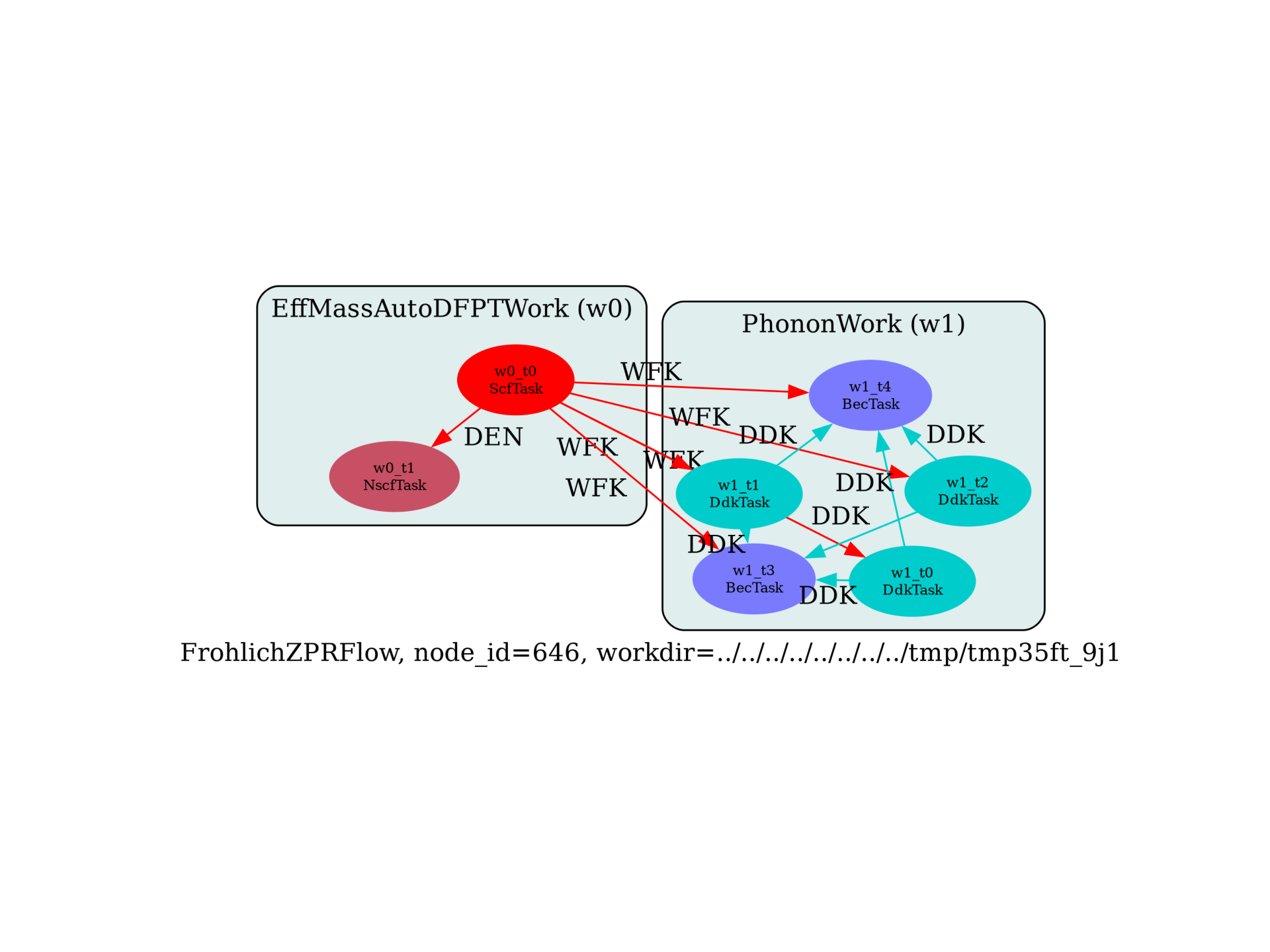# Estimate the ZPR at band edges with the generalized Frohlich model¶

Flow to estimate the zero-point renormalization at the band edges using the generalized Frohlich model. The flow uses DFPT to compute the effective masses at the band edges (automatically detected by performing a NSCF run with a k-path), BECS, eps_inf and phonon frequencies at Gamma

import sys
import os
import abipy.data as abidata
import abipy.abilab as abilab
import abipy.flowtk as flowtk

def make_scf_input(usepaw=0):
"""Returns the GS input file"""
# Here we use parameters similar to https://docs.abinit.org/tests/v8/Input/t57.in
pseudos = abidata.pseudos("Ca.psp8", "O.psp8")

structure = dict(
acell=3 * [9.136],
xred=[
0.0000000000, 0.0000000000, 0.0000000000,
0.5000000000, 0.5000000000, 0.5000000000],
rprim=[
0  , 0.5, 0.5,
0.5, 0  , 0.5,
0.5, 0.5, 0],
typat=[1, 2],
natom=2,
ntypat=2,
znucl=[20, 8],
)

scf_input = abilab.AbinitInput(structure=structure, pseudos=pseudos)

scf_input.set_vars(
nband=12,
nbdbuf=2,
diemac=6,
ecut=30,               # Underconverged ecut.
#ecut=15,
nstep=100,
tolvrs=1e-16,
kptrlatt=[-2,  2,  2,  # In cartesian coordinates, this grid is simple cubic
2, -2,  2,
2,  2, -2],
)

return scf_input

def build_flow(options):
# Set working directory (default is the name of the script with '.py' removed and "run_" replaced by "flow_")
if not options.workdir:
options.workdir = os.path.basename(sys.argv).replace(".py", "").replace("run_", "flow_")

# Build the SCF input.
scf_input = make_scf_input()

# Build the flow.
from abipy.flowtk.effmass_works import FrohlichZPRFlow
flow = FrohlichZPRFlow.from_scf_input(options.workdir, scf_input, ndivsm=4, tolwfr=1e-16,
manager=options.manager)

return flow

# This block generates the thumbnails in the Abipy gallery.
# You can safely REMOVE this part if you are using this script for production runs.
__name__ = None
import tempfile
options = flowtk.build_flow_main_parser().parse_args(["-w", tempfile.mkdtemp()])
build_flow(options).graphviz_imshow()

@flowtk.flow_main
def main(options):
"""
This is our main function that will be invoked by the script.
flow_main is a decorator implementing the command line interface.
Command line args are stored in options.
"""
return build_flow(options)

if __name__ == "__main__":
sys.exit(main())Out:

/Users/gmatteo/git_repos/pymatgen/pymatgen/util/plotting.py:550: UserWarning: Matplotlib is currently using agg, which is a non-GUI backend, so cannot show the figure.
plt.show()


Run the script with:

run_frohlich_zpr -s

Total running time of the script: ( 0 minutes 0.845 seconds)

Gallery generated by Sphinx-Gallery